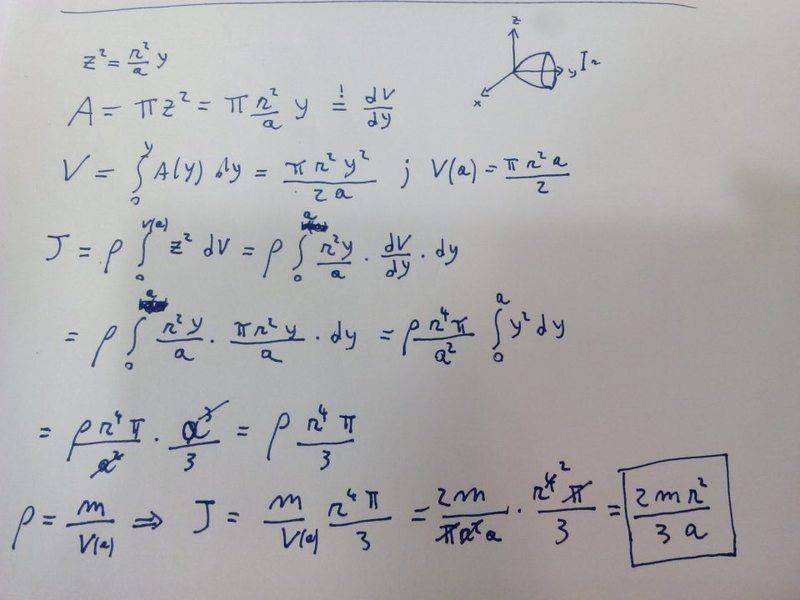Is my procedure correct to compute this moment of inertia J?

Looking at the image below, we have the function

$z^2=\frac{r^2}{a} y$

revolving about y-axis. We know that y goes from 0 to "a". We also know the mass of the object (of uniform density). Do you think is my procedure correct? Do you get the same result?